/

### Calibrating probability distributions used for risk measurement purposes to market-implied data: 2. Multi-instrument calibration – Section Conclusion

Next Section

2.5          What in practice does this mean in the n-instrument case? Suppose we wish to calibrate to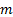different variances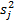(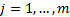) exhibited by instrument baskets described by vectors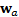, where eachis a vector ofelements, the first element of which is the weight in the basket of the first instrument etc. For example, suppose we have implied volatilities for each instrument in isolation and for an equally weighted portfolio of the instruments.  We would then have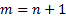calibrations, the firstof which involve weight vectors of the form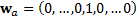(with the’th element of the weight vector being 1, other terms being zero) and the last calibration having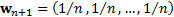. If instead of calibrating to the implied volatility of an equally weighted basket we wished to calibrate to the implied volatility of a market cap weighted index implied volatility then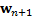would be a vector of index weights.

2.6          The calibrated probability distribution will then have a covariance matrix as follows, where each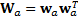is an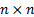dimensional matrix: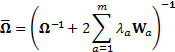subject to thecalibration equations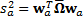.

2.7          As long as this problem is not ill posed (e.g. because there are too many calibrations relative to the number of terms in the covariance matrix, or because there are no feasible solutions to the equations) calibration involves solving a set ofsimultaneous equationsin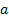unknowns, i.e. the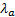.

2.8          An example of such a calibration is set out in the Appendix.

{/CalibratingPriorsToMarketImpliedData2c}